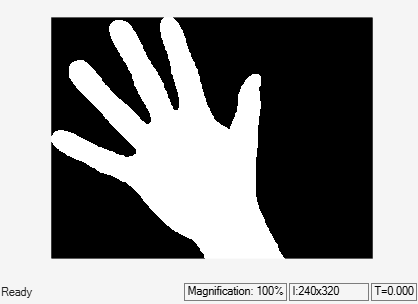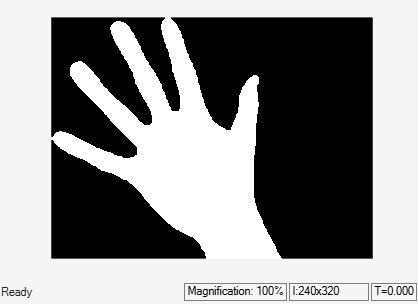Boundary Extraction of Binary Image

This example shows how to extract a boundary from a binary image.

Example Model

modelname = 'ex_blkErosion.slx';
open_system(modelname);The model reads an input binary image using the Image From File block. The Erosion block erodes the input image using the default 4 pixel wide, square structuring element. To extract the boundary of the input image, the model uses the Subtract block to subtract the eroded image from the input image. The Data Type Conversion (Simulink) block converts the data type of the resulting image to Boolean, to match it with the data type of the input image.

Simulate and Display Results

Run the model and visualize the extracted boundary of the input binary image. The model displays the input image, eroded image, and output image using Video Viewer blocks.

sim(modelname);